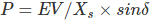# MCQs on Electrical Machines

##### Page 45 of 114. Go to page 1 2 3 4 5 6 7 8 9 10 11 12 13 14 15 16 17 18 19 20 21 22 23 24 25 26 27 28 29 30 31 32 33 34 35 36 37 38 39 40 41 42 43 44 45 46 47 48 49 50 51 52 53 54 55 56 57 58 59 60 61 62 63 64 65 66 67 68 69 70 71 72 73 74 75 76 77 78 79 80 81 82 83 84 85 86 87 88 89 90 91 92 93 94 95 96 97 98 99 100 101 102 103 104 105 106 107 108 109 110 111 112 113 114
01․ The essential condition for parallel operation of two D.C generators is that they have
same KW rating.
same operation r.p.m.
same dropping voltage characteristics.
same percentage regulation.

The following conditions must be satisfied for the parallel operation of DC generator. They are, 1. The terminal voltage of all generators must be same. 2. The polarities of all generators must be same. 3. The prime movers must have the same and stable characteristics from the rotation point of view. 4. The percent voltage drop with respect to the load variation must be same for all generators at all time.

02․ Which of the following load normally needs starting torque more than rated torque?
Blowers.
Conveyers.
Air compressors.
Centrifugal pumps.

The conveyor belts are used to transfer a set of things from one place another place. During running condition, we can’t place any of the materials. So, before starting the conveyor belt, we need to place everything on the conveyor belt. Hence, we need more starting torque to run that load. Basically, in the conveyor belts, three phase slip ring induction motors (or) synchronous motors are used to achieve the high starting torque.

03․ The working principle of transformer depends on
Coulombs law.
Amperes law.
Newtons law.

The transformer is working under the mutual inductance principle. This principle is derived from the Faradays law of electromagnetic induction. Hence, it depends on that principle.

04․ The power developed by salient pole synchronous machine is/are
excitation power only
power due to saliency or reluctance only
both 1 and 2
none of the above

The power developed P = EV/Xd * sinδ + V²/2 * (Xd-Xq)/(Xd*Xq) *sin2δ Where E = Excitation voltage V = Terminal voltage Xd = Direct axis reactance, Xq = Quadrature axis reactance δ = load angle Power due to excitation = EV/Xd * sinδ Power due to saliency or reluctance = V²/2 * (Xd-Xq)/(Xd*Xq) *sin2δ Therefore, the power developed by salient pole synchronous machine contains two components.

05․ In salient pole synchronous machine, if direct axis reactance and quadrature axis reactance is equal, then power developed due to reluctance is
maximum
infinite value
zero
any of the above

The power developed in salient pole synchronous machine P = EV/Xd * sinδ + V²/2 * (Xd - Xq)/(Xd*Xq) *sin2δ Where E = Excitation voltage V = Terminal voltage Xd = Direct axis reactance, Xq = Quadrature axis reactance δ = load angle Power developed due to reluctance = V²/2 * (Xd - Xq)/(Xd*Xq) *sin2δ Therefore, when direct axis reactance Xd is equal to quadrature axis reactance Xq, the power developed due to reluctance is zero.

06․ In salient pole synchronous machine, the reluctance power varies as
sinδ
sin2δ
cosδ
cos2δ

The power developed in salient pole synchronous machine P = EV/Xd * sinδ + V²/2 * (Xd - Xq)/(Xd*Xq) *sin2δ Where E = Excitation value V = Terminal voltage Xd = Direct axis reactance, Xq = Quadrature axis reactance δ = load angle Power due to reluctance = V²/2 * (Xd - Xq)/(Xd*Xq) *sin2δ Therefore, in salient pole synchronous machine, the reluctance power varies as sin2δ.

07․ In salient pole synchronous machine, maximum power occurs at a load angle of
90°
Greater than 90°
less than 90°

The power developed in salient pole synchronous machine P = EV/Xd * sinδ + V²/2 * (Xd-Xq)/(Xd*Xq) *sin2δ Excitation power varies as sinδ and power due to reluctance varies as sin2δ. Maximum excitation power occurs at 90° and maximum power due to reluctance occurs at 45°. Therefore, the maximum power developed in salient pole synchronous machine occurs at load angle less than 90°.

08․ The maximum power developed in cylindrical synchronous machine is occurs at a load angle of
90o.
0o.
Greater than 90o.
less than 90o.

The power developed in cylindrical synchronous machine isMaximum power developed Pmax = EV/Xs Where E = Excitation voltage V = Terminal voltage Xs = Synchronous reactance δ = load angle Therefore, maximum power developed in cylindrical synchronous machine is at a load angle of 90°.

09․ Maximum power developed in a cylindrical synchronous machine depends on
excitation
synchronous reactance